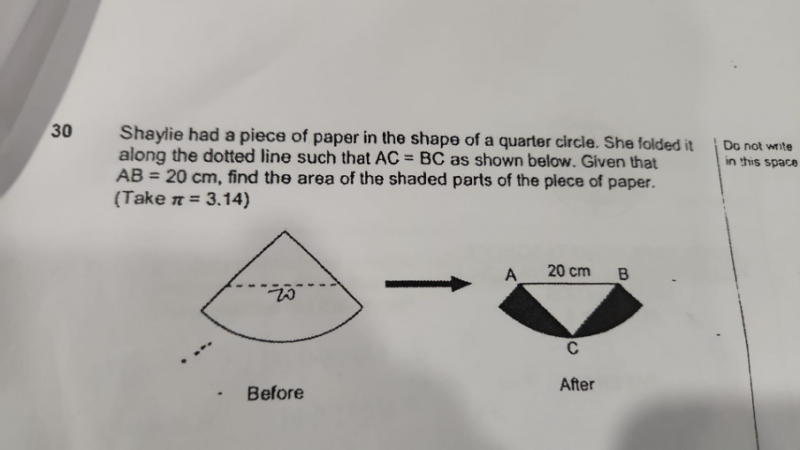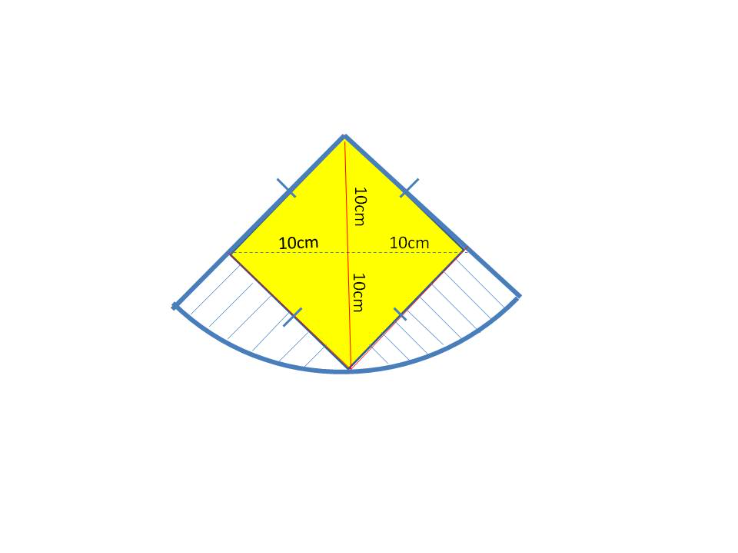# QuestionThank you.From my diagram, you can see that the folded figure is a square because all the sides are equal.

Hence the  two diagonals are 20 cm which means the radius of the quadrant is 20cm.

Area of quadrant = (πr2 ) / 4 =  (3.14 x 20 x 20) / 4 = 314

Area of the square =  (1/2) b x h x 4  = 1/2 x 10 x 10 x 4 = 200   (made up of 4 right angled triangles)

Hence area of shaded part = 314-200 = 114cm2